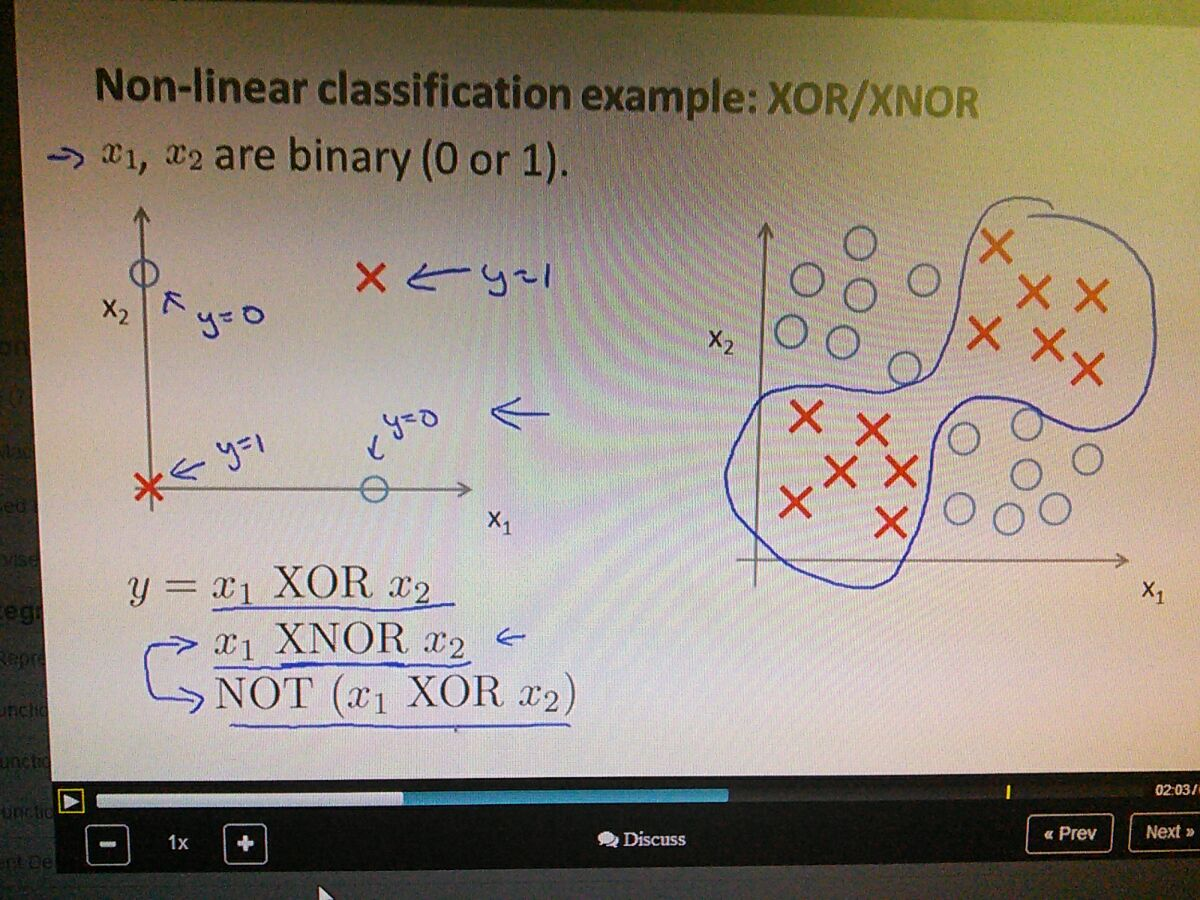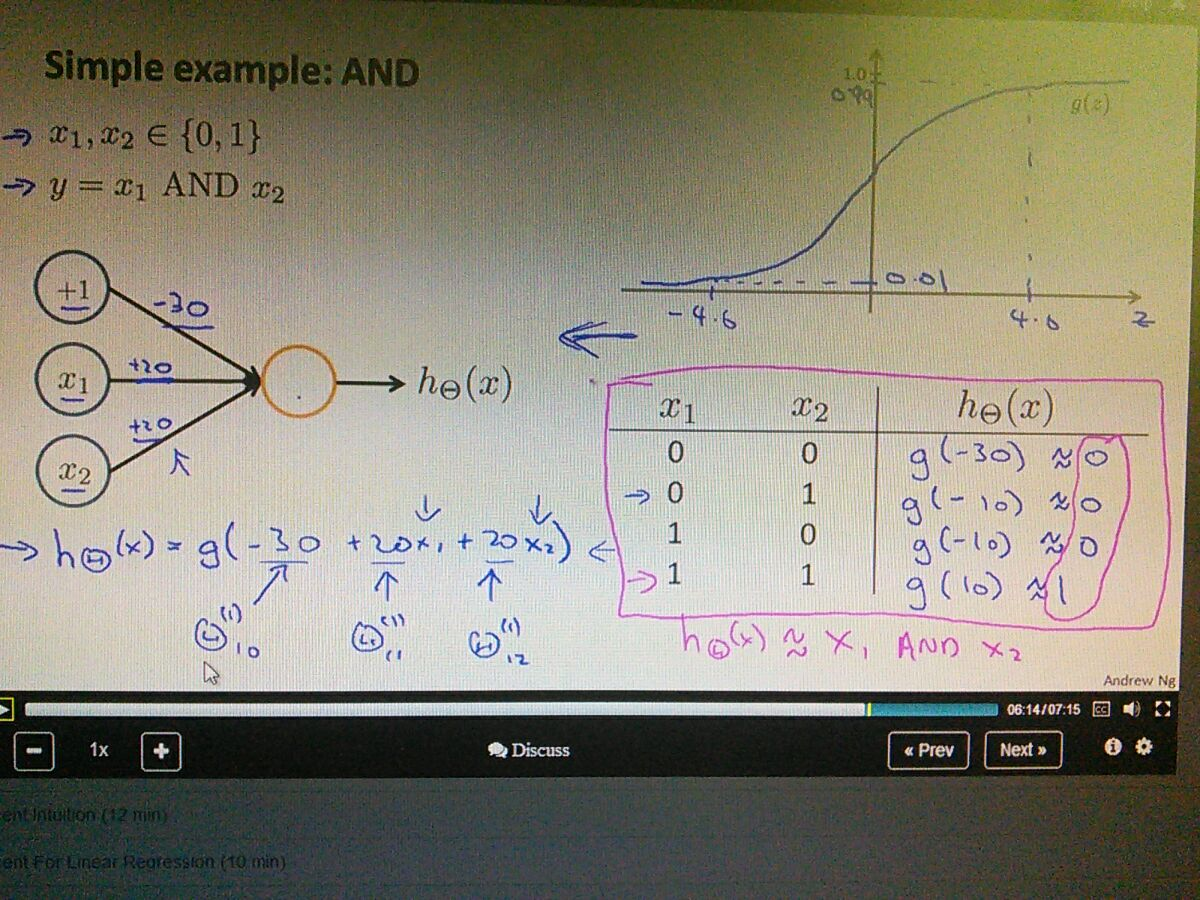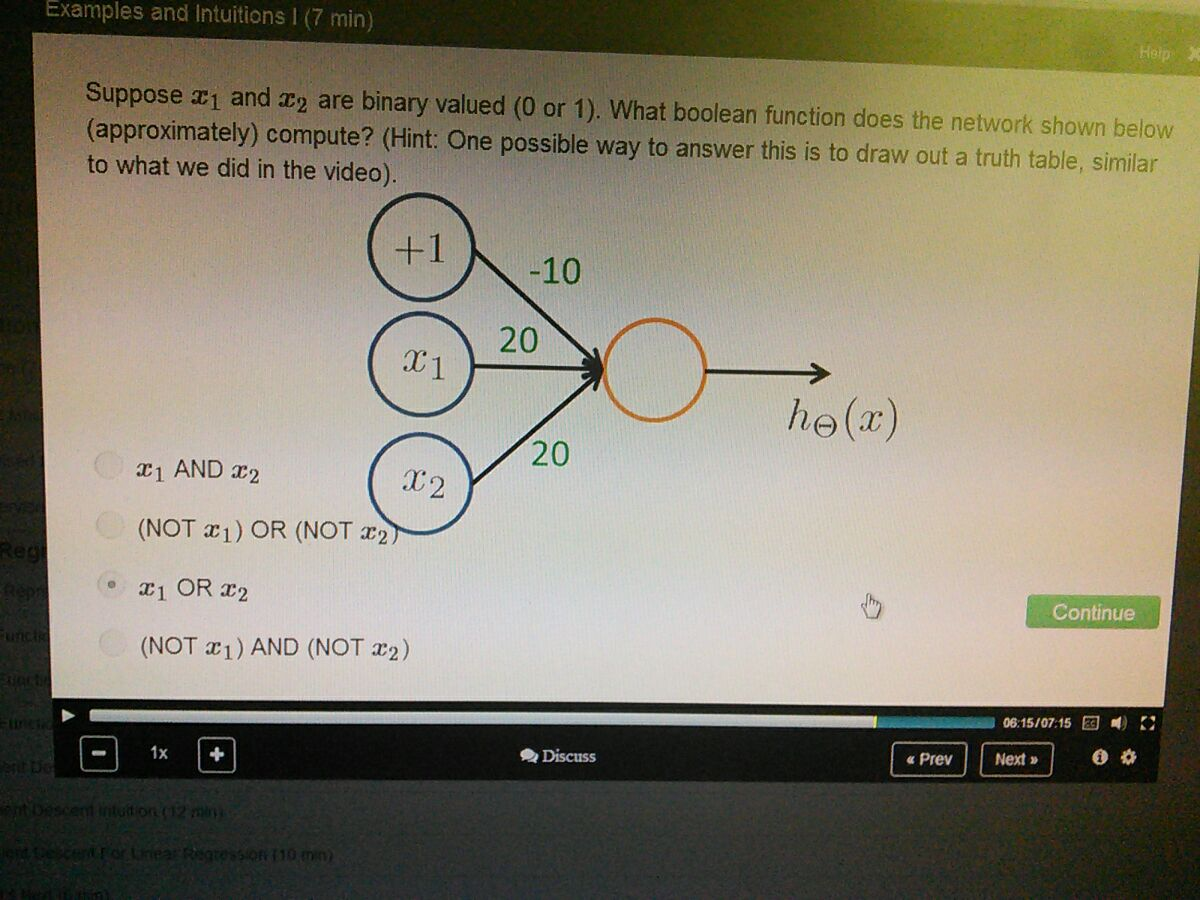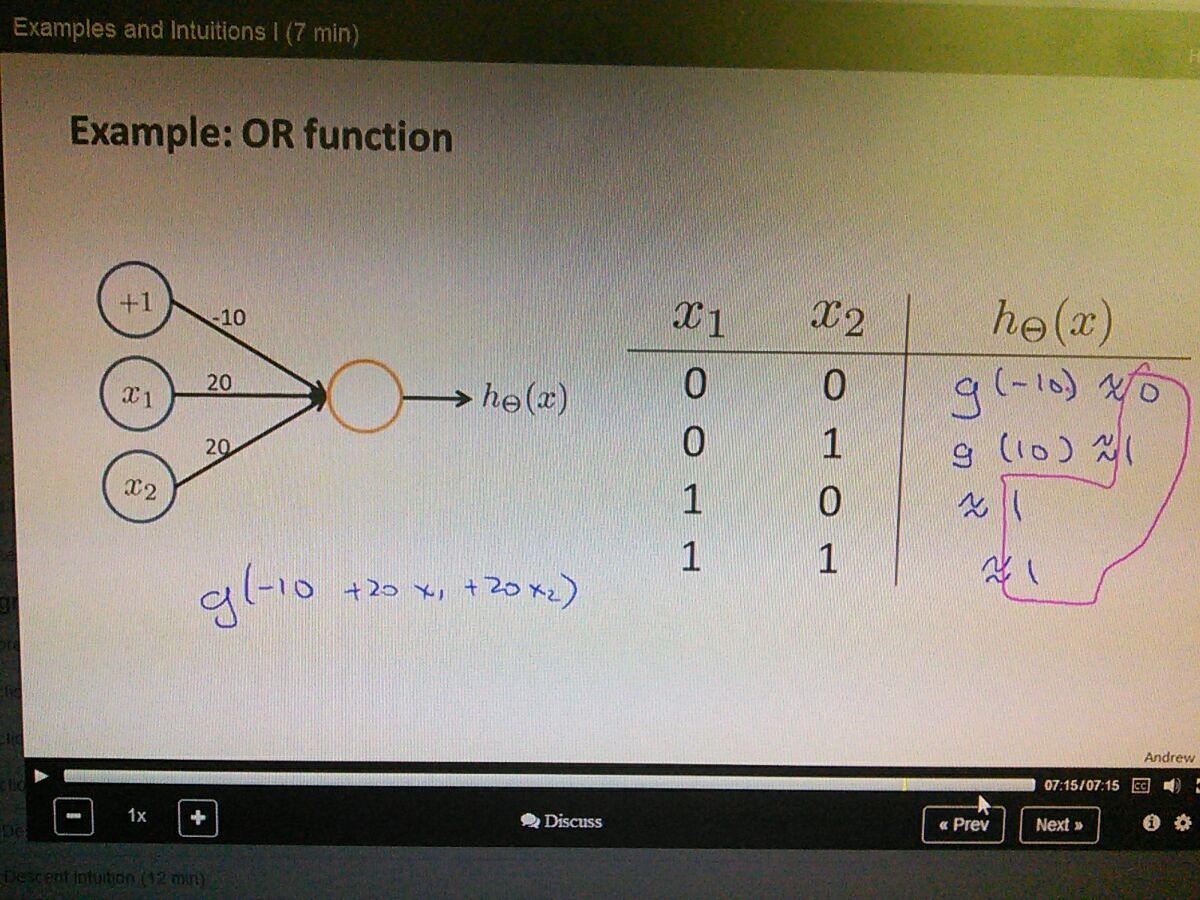give better intuition for neural networks• neural networks to solve xor/xnor• better to add value to the edges of the graph to help better visualize
• the truth table works with disabling(multiplied by zero)
• in the end, the result is the same as AND truth table• 0 0 = -10 (0)
• 0 1 = 10 (1)
• 1 0 = 10 (1)
• 1 1 = 30 (1)
• works like OR truth table• these are neural networks solving logical base truth table function
• next discuss about multiple layer of units solving xor/xnor function